Next: Radiation Losses Up: Relativity and Electromagnetism Previous: Accelerated Charges

# Larmor Formula

Let us transform to the inertial frame in which the charge is instantaneously at rest at the origin at time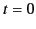. In this frame,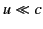, so that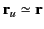and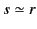for events that are sufficiently close to the origin atthat the retarded charge still appears to travel with a velocity that is small compared to that of light. It follows from the previous section that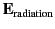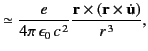(1921)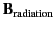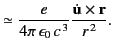(1922)

Let us define spherical polar coordinates whose axis points along the direction of instantaneous acceleration of the charge. It is easily demonstrated that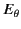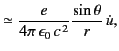(1923)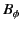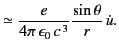(1924)

These fields are identical to those of a radiating dipole whose axis is aligned along the direction of instantaneous acceleration. The radial Poynting flux is given by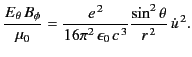(1925)

We can integrate this expression to obtain the instantaneous power radiated by the charge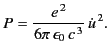(1926)

This is known as Larmor's formula. Note that zero net momentum is carried off by the fields (1925) and (1926).

In order to proceed further, it is necessary to prove two useful theorems. The first theorem states that if a 4-vector field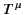satisfies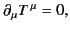(1927)

and if the components ofare non-zero only in a finite spatial region, then the integral over 3-space,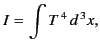(1928)

is an invariant. In order to prove this theorem, we need to use the 4-dimensional analog of Gauss's theorem, which states that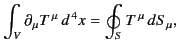(1929)

where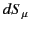is an element of the 3-dimensional surface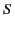bounding the 4-dimensional volume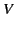. The particular volume over which the integration is performed is indicated in Figure 27. The surfacesand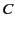are chosen so that the spatial components ofvanish onand. This is always possible because it is assumed that the region over which the components ofare non-zero is of finite extent. The surface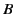is chosen normal to the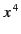-axis, whereas the surface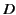is chosen normal to the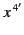-axis. Here, the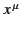and the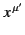are coordinates in two arbitrarily chosen inertial frames. It follows from Equation (1931) that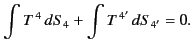(1930)

Here, we have made use of the fact that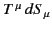is a scalar and, therefore, has the same value in all inertial frames. Because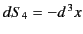and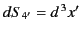it follows that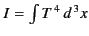is an invariant under a Lorentz transformation. Incidentally, taking the limit in which the two inertial frames are identical, the previous argument also demonstrates that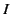is constant in time.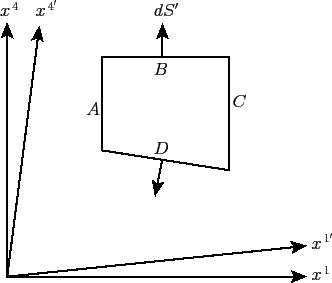The second theorem is an extension of the first. Suppose that a 4-tensor field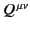satisfies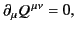(1931)

and has components which are only non-zero in a finite spatial region. Let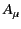be a 4-vector whose coefficients do not vary with position in space-time. It follows that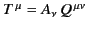satisfies Equation (1929). Therefore,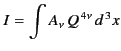(1932)

is an invariant. However, we can write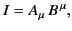(1933)

where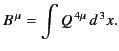(1934)

It follows from the quotient rule that if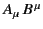is an invariant for arbitrarythen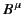must transform as a constant (in time) 4-vector.

These two theorems enable us to convert differential conservation laws into integral conservation laws. For instance, in differential form, the conservation of electrical charge is written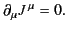(1935)

However, from Equation (1932) this immediately implies that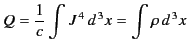(1936)

is an invariant. In other words, the total electrical charge contained in space is both constant in time, and the same in all inertial frames.

Suppose thatis the instantaneous rest frame of the charge. Let us consider the electromagnetic energy tensor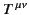associated with all of the radiation emitted by the charge between timesand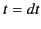. According to Equation (1880), this tensor field satisfies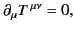(1937)

apart from a region of space of measure zero in the vicinity of the charge. Furthermore, the region of space over whichis non-zero is clearly finite, because we are only considering the fields emitted by the charge in a small time interval, and these fields propagate at a finite velocity. Thus, according to the second theorem,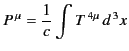(1938)

is a 4-vector. It follows from Section 12.22 that we can write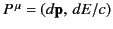, where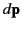and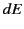are the total momentum and energy carried off by the radiation emitted between timesand, respectively. As we have already mentioned,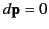in the instantaneous rest frame. Transforming to an arbitrary inertial frame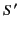, in which the instantaneous velocity of the charge is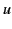, we obtain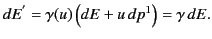(1939)

However, the time interval over which the radiation is emitted inis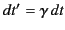. Thus, the instantaneous power radiated by the charge,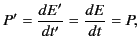(1940)

is the same in all inertial frames.

We can make use of the fact that the power radiated by an accelerating charge is Lorentz invariant to find a relativistic generalization of the Larmor formula, (1928), which is valid in all inertial frames. We expect the power emitted by the charge to depend only on its 4-velocity and 4-acceleration. It follows that the Larmor formula can be written in Lorentz invariant form as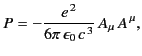(1941)

because the 4-acceleration takes the form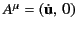in the instantaneous rest frame. In a general inertial frame,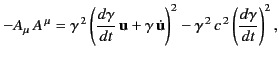(1942)

where use has been made of Equation (1726). Furthermore, it is easily demonstrated that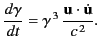(1943)

It follows, after a little algebra, that the relativistic generalization of Larmor's formula takes the form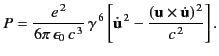(1944)Next: Radiation Losses Up: Relativity and Electromagnetism Previous: Accelerated Charges
Richard Fitzpatrick 2014-06-27